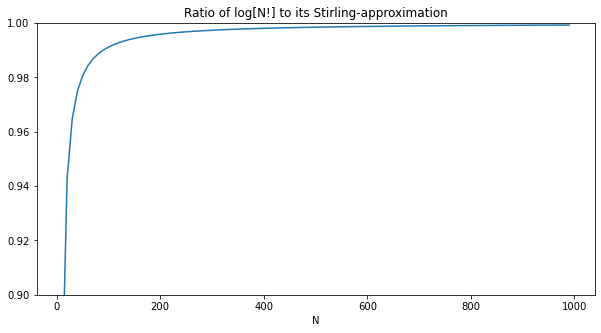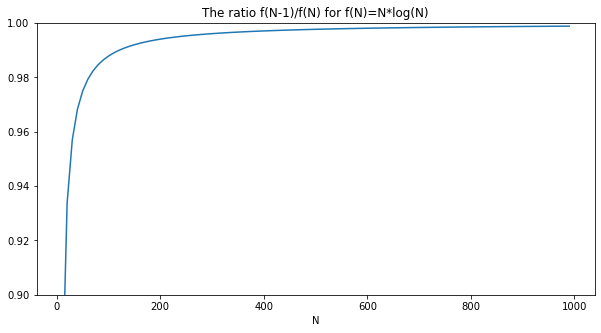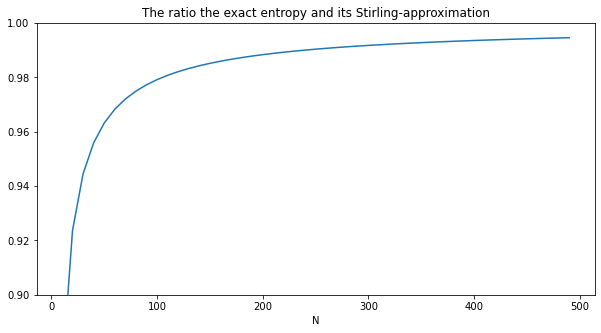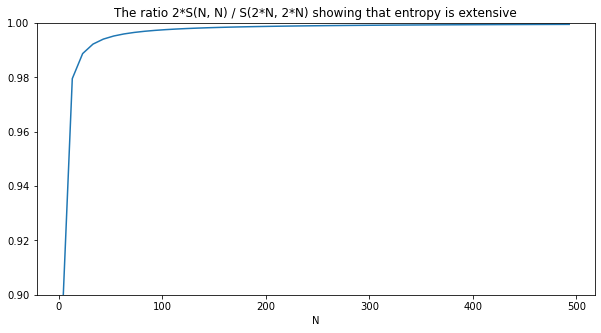# Entropy of an ideal gas with coarse-graining

Marton Trencseni - Fri 19 November 2021 - Physics

## Introduction

An ideal gas is a model of a real physical gas where we make some simplifications. We model the gas as hard billiard balls, bouncing off the walls of the container and each other in perfectly elastic collisions. In classical physics, momentum is always conserved, in elastic collisions kinetic energy is also conserved (ie. kinetic energy never gets transfered to some other form of energy ). Here I will consider a monoatomic ideal gas, ie. all the gas is made up of one type of billiard ball.

 In inelastic collisions, kinetic energy is lost as energy is converted to heat energy. This wouldn't make sense here, since we are using the billiard balls at the micro level to model heat and temperature at the macro level.

Entropy is the function of the probabilities in a random variable. If we assume that all outcomes are equally likely, so $p_i = p = 1/N$, then the expression for the entropy becomes $H = log[N]$.

Let's start with a naive (and wrong) thought experiment to get to entropy. Let's assume we have a container, with 1 particle in it. The particle has some velocity and is bouncing around the container. Let's compute the entropy due to the position of the particle.

Let's start with the simplest possible coarse-graining, and split the container into 2 bins (A and B), so the ball is either in A or B. With this coarse-graining, the entropy of the system is 1 bit. Now, if we imagine 2 of these systems sitting next to each other, but separated by a thin wall, the entropy will be 2 bits. As long as the wall is there, the systems like independent random variables, so the joint entropy H(X, Y) = H(X) + H(Y). What if we now remove the wall? We will have 2 balls in the system, and keeping the physical size of the coarse graining, there are now 4 parts. In total, we can put 2 identical balls into 4 bins $4+3+2+1=10$ ways, so the entropy is $log_2=3.3$ bits. So the entropy of the combined system with the wall removed is greater than the entropy of the sum of the individual systems, because the balls can now occupy just the left side or just the right side of the system, a state that was not present before.

From a physical point of view, this is where our thought experiment fails. If we put two identical containers of monatomic gas (identical atoms, pressure, temperature, density) next to each other, remove the barrier, and let them mix, then effectively nothing happens, so intensive properties (like pressure, temperature, chemical potential) remain the same and extensive properties (like volume, entropy and particle number) are added (they are additive). In the above example, we'd expect the combined system to have 2 bits of entropy (at this level of coarse-graining).

The problem is simple: the ideal gas model of thermodynamics only works if $N$, the number of particles is very large, and $N=1$ is not large enough. So let's consider a lot of particles.

## Notation

In physics, when we deal with entropy, there is some minor differences in notation:

• we use $S$ instead of $H$ to denote entropy
• after Ludwig Boltzmann, we use $W$ instead of $N$ to denote the total number of possible outcomes (W as in "ways things can happen")
• we the $ln=log_e$ instead of $log_2$

## Marbles and bins

Let's generalize the above naive approach, and imagine $N$ particles and a coarse-graining of volume so that we have $M$ bins of equal volume. Given $N$ indistinguishible marbles, how many $W$ ways can we put them into $M$ distinguishible bins? The answer, using combinatorics, is:

$W(N, M) = \frac{(N + M - 1)!}{N!(M-1)!}$

You can double-check on paper that in the case of the above toy model, $W(2, 4) = 10$.

In a physical system, assuming all microscopic states are equally likely, entropy scales like $S \approx log[W]$. With this, we can revisit the above situation of putting two containers of ideal gas next to each other. The question is:

$S(W(2N, 2M)) \stackrel{?}{=} 2 S(W(N, M))$

## Stirling's approximation

The last tool we need is Stirling's approximation. For large $N$:

$ln[N!] \approx N ln[N] - N$

Using basic properties of the logarithm ($log[ab] = log[a] + log[b]$ and $log[\frac{a}{b}] = log[a] - log[b]$):

$S(W(2N, 2M)) = ln[\frac{(2N + 2M - 1)!}{(2N)!(2M-1)!}] = ln[(2N + 2M - 1)!] - ln[2N!] - ln[(2M-1)!]$.

Then, using Stirling's approximation:

$S(W(2N, 2M)) = (2N + 2M - 1) ln[2N + 2M - 1] - (2N + 2M - 1) - 2N ln[2N] + 2N - (2M-1) ln[2M-1] + (2M-1)$

The non-log parts cancel out, so:

$S(W(2N, 2M)) = (2N + 2M - 1) ln[2N + 2M - 1] - 2N ln[2N] - (2M-1) ln[2M-1]$.

For large N, we can approximate $(N-1) ln[N-1] \approx N ln[N]$, so:

$S(W(2N, 2M)) \approx (2N + 2M) ln[2(N + M)] - 2N ln[2N] - 2M ln[2M]$

Now, we can break the logs out, like $ln[2N] = ln + ln[N]$. The terms multiplied with $ln$ just cancel out, so:

$S(W(2N, 2M)) \approx 2(N + M) ln[N + M] - 2N ln[N] - 2M ln[M] = 2 ((N + M) ln[N + M] - N ln[N] - M ln[M]) \approx 2 S(W(N, M))$.

So, for large N, the coarse-grained entropies are additive (approximately)! It we consider something like a liter of gas, it will have $N \approx 10^{22}$, so these approximations hold to very high precision.

## Numerical checks

The code shown below is up on Github. First, let's check Stirling's approximation for large N: $ln[N!] \approx N ln[N] - N$

# numerical verification of Stirling's approximation for large N

def log_factorial(N):
# compute log(N!) as a sum, so we can compute if for large N
# using the identity log(a*b) = log(a) + log(b)
# this is not an approximation, aside from floating point issues
sum = 0
for i in range(1, N+1):
sum += log(i)
return sum

def stirling(N):
return N*log(N)-N

xs, ys = [], []
for N in range(10, 1000, 10):
xs.append(N)
ys.append(stirling(N)/log_factorial(N))
plot(xs, ys, title='Ratio of log[N!] to its Stirling-approximation', xlabel='N')Next, let's verify that $(N-1) ln[N-1] \approx N ln[N]$:

# numerical verification that (N-1)*log(N-1) ~ N*log(N) for large N

def f(N):
return N*log(N)

xs, ys = [], []
for N in range(10, 1000, 10):
xs.append(N)
ys.append(f(N-1)/f(N))
plot(xs, ys, title='The ratio f(N-1)/f(N) for f(N)=N*log(N)', xlabel='N')Next, let's verify that the exact entropy S and its Stirling-approximated are the same for large N:

# numerical verification that the exact entropy S and its
# Stirling-approximated are the same for large N

def W(N, M):
return factorial(N+M-1) / (factorial(N)*factorial(M-1))

def S(N, M):
return log(W(N,M))

def S_stirling(N, M):
return (N+M-1)*log(N+M-1) - (M-1)*log(M-1) - N*log(N)

xs, ys = [], []
for N in range(10, 500, 10):
xs.append(N)
ys.append(S(N, N)/S_stirling(N, N))
plot(xs, ys, title='The ratio the exact entropy and its Stirling-approximation', xlabel='N')Finally, let's verify our final result that the entropy $S$ is extensive:

# numerical verification that entropy is extensive

def S_stirling(N, M):
return (N+M-1)*log(N+M-1) - (M-1)*log(M-1) - N*log(N)

xs, ys = [], []
for N in range(3, 500, 10):
xs.append(N)
ys.append(2*S_stirling(N, N) / S_stirling(2*N, 2*N))
plot(xs, ys, title='The ratio 2*S(N, N) / S(2*N, 2*N) showing that entropy is extensive', xlabel='N')## Next steps

The above formula for entropy cannot be physical, since it depends on how to decide to coarse grain the volume of the gas ($M$). Also, it doesn't take into account the velocity of the particles. The next step, coming in the next article, is to take make physical arguments and compute an expression for $W$ that takes into account the velocities, parametrize using the kinetic energy of the system and derive the Sackur–Tetrode equation. Once we have that, we can also derive the ideal gas equation of state $pV = NkT$.